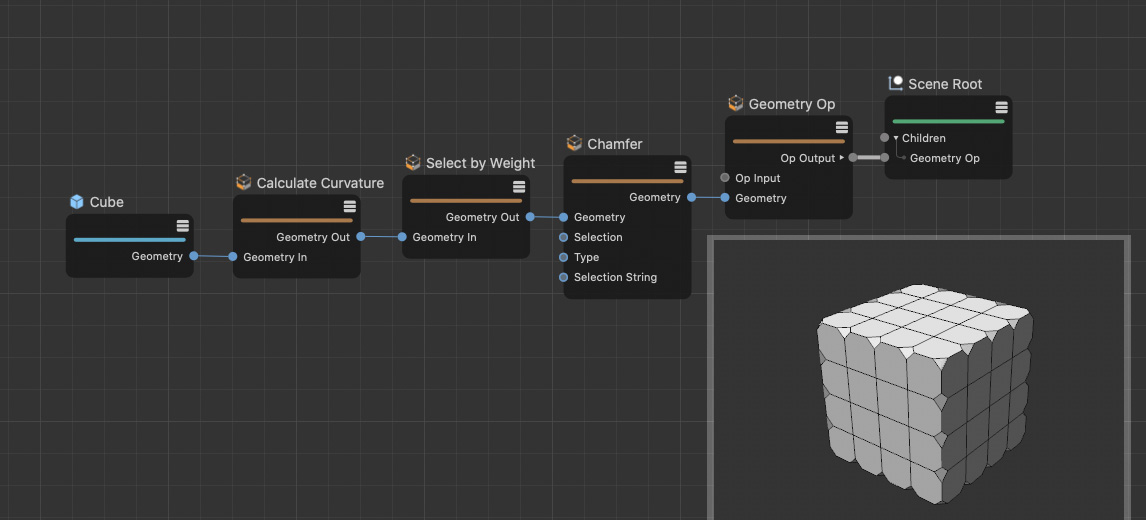Calculate Curvature

This Node adds a weight map to a geometry. The values of the weight map range between 0% and 100% and indicate the angles between the neighboring point normals.The Node calculates weight values for vertices based on the surrounding surface normal. In this example the weight information is converted to a selection using the Select by Weight Node.